## Main

Heavy ions are collided at very high energies at the CERN Large Hadron Collider (LHC) to study matter at extremely high temperatures and densities. Under these conditions heavy-ion collisions are a copious source of matter and anti-matter particles and thus are suitable for an experimental investigation of their properties such as mass and electric charge. In relativistic heavy-ion collisions, nuclei and corresponding anti-nuclei are produced with nearly equal rates7. Their yields have been measured at the Relativistic Heavy Ion Collider (RHIC) by the STAR (ref. 8) and PHENIX (ref. 9) experiments and at the LHC by the ALICE (ref. 4) experiment. So far, the heaviest anti-nucleus which has been observed7 is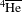(anti-α); meanwhile, for lighter nuclei and anti-nuclei, which are more copiously produced, a detailed comparison of their properties is possible. This comparison represents an interesting test of CPT symmetry in an analogous way as done for elementary fermions10,11 and bosons12, and for QED (refs 13, 14) and QCD systems1,2,15,16,17 (a particular example for the latter being the measurements carried out on neutral kaon decays18), with different levels of precision which span over several orders of magnitude. All these measurements can be used to constrain, for different interactions, the parameters of effective field theories that add explicit CPT violating terms to the standard model Lagrangian, such as the standard model extension19 (SME).

The measurements reported in this paper are based on the high-precision tracking and identification capabilities of the ALICE experiment20. The main detectors employed in this analysis are the ITS (inner tracking system)21 for the determination of the interaction vertex, the TPC (time projection chamber)22 for tracking and specific energy loss (dE/dx) measurements, and the TOF (time of flight)23 detector to measure the time tTOF needed by each track to traverse the detector. The combined ITS and TPC information is used to determine the track length (L) and the rigidity (p/z, where p is the momentum and z the electric charge in units of the elementary charge e) of the charged particles in the solenoidal 0.5 T magnetic field of the ALICE central barrel (pseudo-rapidity |η| < 0.8). On the basis of these measurements, we can extract the squared mass-over-charge ratio μTOF2 ≡ (m/z)TOF2 = (p/z)2 [(tTOF/L)2 − 1/c2]. The choice of this variable is motivated by the fact that μ2 is directly proportional to the square of the time of flight, allowing to better preserve its Gaussian behaviour.

The high precision of the TOF detector, which determines the arrival time of the particle with a resolution of 80 ps (ref. 20), allows us to measure a clear signal for (anti-)protons, (anti-)deuterons and (anti-)3He nuclei over a wide rigidity range (1 < p/|z| < 4 GeV/c). The main source of background, which is potentially of the same order of the signal, arises from tracks erroneously associated to a TOF hit. To reduce this contamination, a 2σ cut (where σ is the standard deviation) around the expected TPC dE/dx signal is applied. Such a requirement strongly suppresses (to below 4%) this background for rigidities below p/|z| < 2.0 GeV/c for (anti-)deuterons and for all rigidities for (anti-)3He (to below 1%). For each of the species under study, the mass is extracted by fitting the mass-squared distributions in narrow p/|z| and η intervals, using a Gaussian with a small exponential tail that reflects the time signal distribution of the TOF detector. Examples of the mass-squared distributions for (anti-)deuterons and (anti-)3He candidates are reported in Fig. 1 in selected rigidity intervals.Figure 1: Examples of squared mass-over-charge ratio distributions in selected rigidity intervals.

Using mass differences, rather than absolute masses, allows us to reduce the systematic uncertainties related to tracking, spatial alignment (affecting the measurement of the track momentum and length) and time calibration. Despite that, residual effects are still present, due to imperfections in the detector alignment and the description of the magnetic field, which can lead to position-dependent systematic uncertainties. In terms of relative uncertainties, the ones affecting the measurement of the momentum are the largest and independent of the mass, and are the same for all positive (negative) particles in a given momentum interval. It is therefore possible to correct the (anti-)deuteron and the (anti-)3He masses by scaling them with the ratio between the (anti-)proton masses recommended by the PDG (particle data group)24 (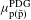) and the ones measured in the analysis presented here (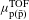), namely,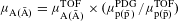. These correction factors, which depend on the rigidity, deviate from unity by at most 1%. Conversely, systematic effects connected to the track-length measurement are mass dependent and cannot be completely accounted for using the above correction. However, they are expected to be symmetric for positive and negative particles when inverting the magnetic field. Any residual asymmetry is therefore indicative of remaining systematic uncertainties related to the detector conditions. To estimate them, and keep these effects under control, both nuclei and anti-nuclei measurements are performed for two opposite magnetic field configurations and then averaged. Their half-difference is taken as the estimate of this systematic uncertainty. Other sources of systematic uncertainties are evaluated by varying energy loss corrections applied to the reconstructed momentum, the range and the shape of the background function assumed in the fit of the mass-squared distributions and the track selection criteria. In particular, TPC dE/dx cuts are varied between one and four standard deviations to probe the sensitivity of the fit results on the residual background, and a tracking quality cut on the distance of closest approach of the track to the vertex is varied to evaluate the influence of secondary particles on the measurement. The sources of systematic uncertainties are found to be fully correlated among all the rigidity intervals, except for those due to the fit procedure and the TPC selection criteria, where the uncertainties are uncorrelated. For deuterons and anti-deuterons, the largest relative systematic uncertainties on Δμ/μ come from the detector alignment (0.7 × 10−4), the TPC selection criteria (0.7 × 10−4) and the secondaries (1.0 × 10−4). For 3He and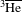, they come from the energy loss corrections (0.7 × 10−3), the fit procedure (0.5 × 10−3) and the TPC selection criteria (0.4 × 10−3).

The (anti-)deuteron and (anti-)3He masses are measured as the peak position of the fitting curves of the mass-squared distribution. The mass-over-charge ratio differences between the deuteron and 3He and their respective anti-particle are then evaluated as a function of the rigidity of the track, as shown in Fig. 2. The measurements in the individual rigidity intervals are combined, taking into account statistical and systematic uncertainties (correlated and uncorrelated), and the final result is shown in the same figure with one and two standard deviation uncertainty bands. The measured mass-over-charge ratio differences are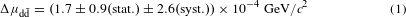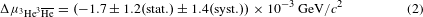corresponding to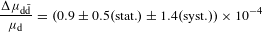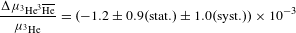where μd and ${\mu }_{{3}_{\text{He}}}$ are the values recommended by CODATA (ref. 25). The mass-over-charge differences are compatible with zero within the estimated uncertainties, in agreement with CPT invariance expectations.Figure 2: d– (top) and 3He– (bottom) mass-over-charge ratio difference measurements as a function of the particle rigidity.

Given thatand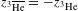as for the proton and anti-proton1,2, the mass-over-charge differences in equations (1) and (2) and the measurement of the mass differences between proton and anti-proton1,2 and between neutron and anti-neutron15,16 can be used to derive the relative binding energy differences between the two studied particle species. We obtainwhere ɛA = Zmp + (AZ)mnmA, with mp and mn being the proton and neutron mass values recommended by the PDG (ref. 24) and mA the mass value of the nucleus with atomic number Z and mass number A, recommended by CODATA (ref. 25). This quantity allows one to explicitly isolate possible violations of the CPT symmetry in the (anti-)nucleon interaction from that connected to the (anti-)nucleon masses, the latter being constrained with a precision of 7 × 10−10 for the proton/anti-proton system1,2. Our results and the comparisons with previous mass difference measurements for (d–) (refs 26, 27) and (3He–) (ref. 28), as well as binding energy measurements for (d–) (refs 29, 30), are reported in Fig. 3.Figure 3: Measurements of the mass-over-charge ratio and binding energies differences for d– and 3He–.

We have shown that the copious production of (anti-)nuclei in relativistic heavy-ion collisions at the LHC represents a unique opportunity to test the CPT invariance of nucleon–nucleon interactions using light nuclei. In particular, we have measured the mass-over-charge ratio differences for deuterons and 3He. The values are compatible, within uncertainties, with zero and represent a CPT invariance test in systems bound by nuclear forces. The results reported here (Fig. 3, left) represent the highest precision direct measurements of mass differences in the sector of nuclei and they improve by one to two orders of magnitude analogous results originally obtained more than 40 years ago26,27,28, and precisely 50 years ago for the anti-deuteron26,27. Remarkably, such an improvement is reached in an experiment which is not specifically dedicated to test the CPT invariance in nuclear systems. In the forthcoming years the increase in luminosity and centre-of-mass energy at the LHC will allow the sensitivity of these measurements to be pushed forwards, and possibly extend the study to (anti-)4He. Given the equivalence between mass and binding energy differences, our results also improve (Fig. 3, right) by a factor two the constraints on CPT invariance inferred by existing measurements29,30 in the (anti-)deuteron system. The binding energy difference has been determined for the first time in the case of (anti-)3He, with a relative precision comparable to that obtained in the (anti-)deuteron system.